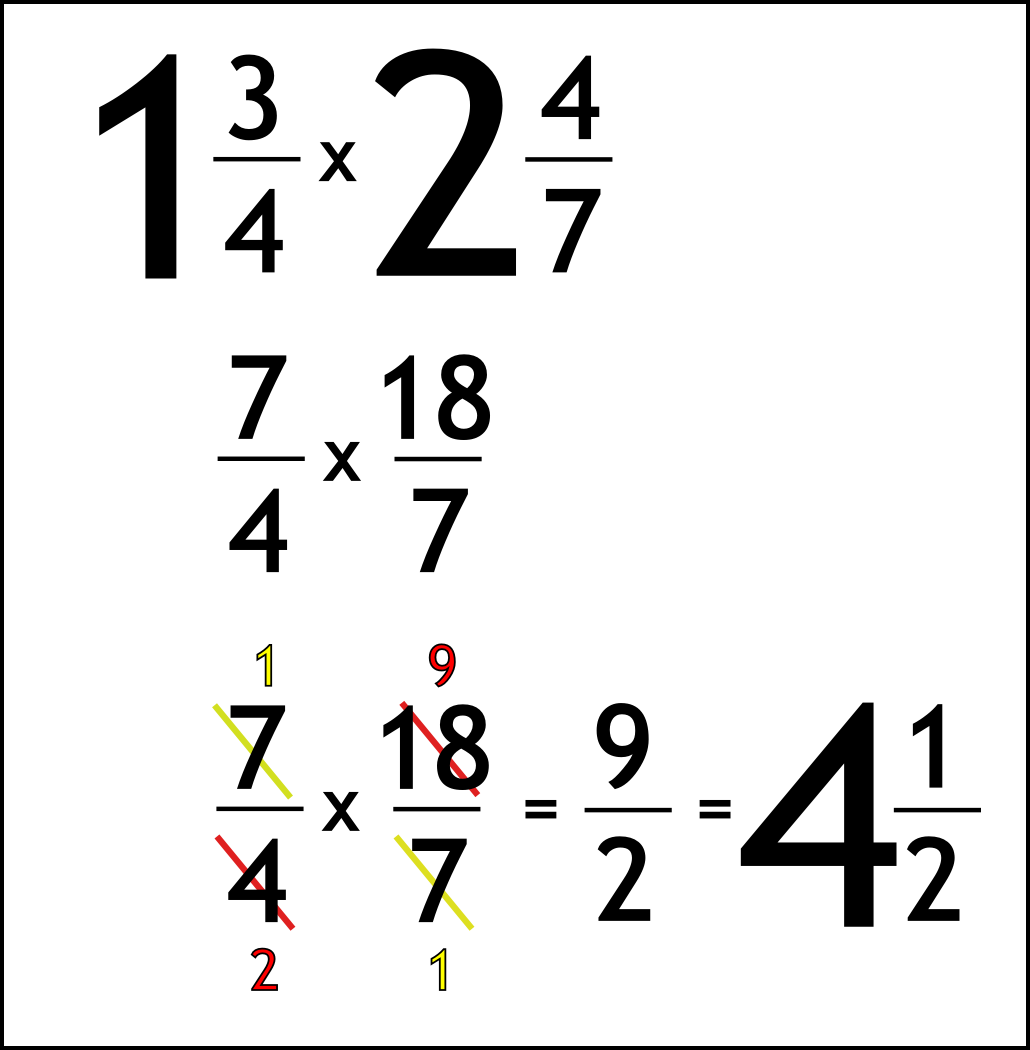The Singing Hedgehog Guide to:
Multiplying Fractions 2

When multiplying mixed numbers we must first turn them into top-heavy fractions.

Once we have only fractions we just do the rule:

IT'S A CROSS SO CROSS THINGS OUT!

Here is another example:Write out the question

Turn mixed into top-heavy
1 times 4 is 4 add 3 gives 7
2 times 7 is 14 add 4 gives 18

Find common factors (numbers to divide by)

7 and 7 are both divisible by 7 to leave 1 and 1

4 and 18 are both divisible by 2 to leave 2 and 9

Multiply across
Turn top-heavy back to mixed
2 into nine goes 4 with 1 left over

next page

fractions index